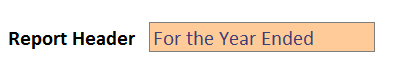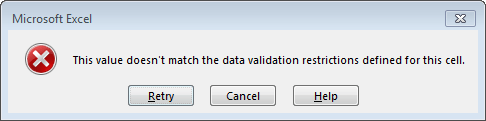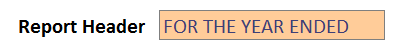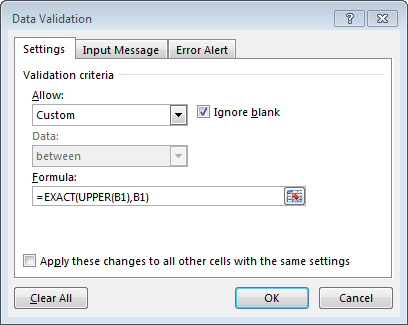# Data Validation with ALL CAPSIn recent back-to-back webinars, two different attendees asked how to check to see if a user entered a text string with all caps. Specifically, they asked how to set up a data validation rule to determine if all letters are capitalized. So, I figured I would write a quick post about the technique.

## Objective

Let’s just take a moment to confirm our goal here. Our goal is to apply a data validation rule to a cell that tests to see if the user entered the value in ALL CAPS.

So, if the user enters a text string with a mix of upper and lower case letters into the input cell, we want Excel to display an error alert dialog. For example, say the user enters the value “For the Year Ended” as shown below.We would want Excel to display an error alert, like the one shown below.But, the user shouldn’t get the error if the value is entered in UPPER case, as shown below.This can be accomplish by creating a custom data validation rule. Let’s jump into the details now.

## Details

We’ll just walk through the steps together.

1) The first step is to select the input cell! After selecting the input cell, make a note of its cell reference, such as B1 for example.

Note: You will be applying data validation to the input cell, and will use its cell reference in the data validation formula below.

2) Next, open the Data Validation dialog by clicking the Data > Data Validation ribbon icon. In the resulting dialog box, select Custom as shown below.3) Then, we need to enter the formula into the dialog’s Formula field. Essentially, we are going to ask Excel to see if the value in the input cell is equal to the upper case version of the input cell. If they are, then the user entered the value in upper case. If not, the user did not enter it in all upper case, and so we need the error alert.

We can accomplish this by using the UPPER function (which converts a text string to upper case) and the EXACT function (which will compare the two values to see if they are the same). Assuming the input cell is B1, we would enter:

`=EXACT(UPPER(B1),B1)`

This is shown in the dialog below.Note: update the formula to refer to your input cell if not B1.

This formula basically returns TRUE when the upper case version of B1 is exactly the same as B1. If not, it returns FALSE, triggering the error alert.

If you have any other fun custom data validation rules, please share by posting a comment below…thanks!

## Resources

Get a quick email notice when a new Excel article is available

• This field is for validation purposes and should be left unchanged.1.susan schiavone

Thanks, Jeff!

1.jefflenning Post author

Welcome 🙂

2.Lisa

Took me a minute using it to realize what it does, is there a way to do the opposite? Make sure it is all proper case? instead of all caps?

1.jefflenning Post author

Yes, indeed! You can use the PROPER function instead of UPPER. You could also use LOWER to test for lower case.

1.Lisa
1.jefflenning Post author
3.Kylie Moore